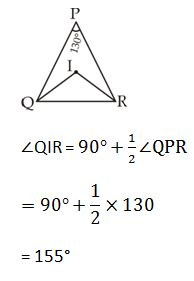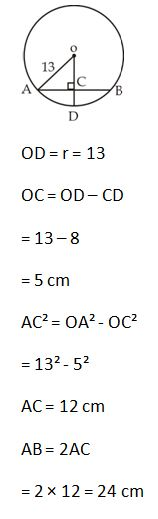# SSC Quantitative Aptitude Questions (Day-52)

Dear Aspirants, you can find the Quantitative Aptitude questions with detailed explanations for the SSC exams. Nowadays the competitive level of the exam has been increasing consistently. Due to the great demand for the government job, the level of the toughness reached greater. Candidates have to enhance the preparation process in order to drive in the right path. It doesn’t need to clear the prescribed cutoff. You must have to score good marks more than the cut off marks to get into the final provisional list. Here we have updating the Quantitative Aptitude questions with detailed explanations on a daily basis. You can practice with us and measure your level of preparation. According to that you can sculpt yourself in a proper way. SSC aspirants kindly make use of it and grab your success in your career.

Start Quiz

1) If x2 – 7x + 1 = 0, then what is the value of x+1/x?

(a) 7

(b) 3

(c) 51

(d) 47

2) If x2 – 2
10x+ 1=0, then what is the value of x –1/x?

(a) 4

(b) 6

(c) 3

(d) 5

3)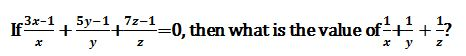(a) -3

(b) 0

(c) 15

(d) 21

4) If x2 – 3x + 1 = 0, then what is the value of x4 +
1/x4 ?

(a) 11

(b) 18

(c) 47

(d) 51

5)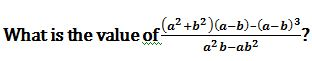(a) 0

(b) 1

(c) -1

(d) 2

6) In the given figure, O is the center of the circle,
PQR= 100o and STR = 105o. What is the value (in degrees) of ∠OSP?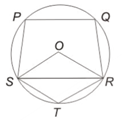(a) 95

(b) 45

(c) 75

(d) 65

7) In the given figure, EF = CE = CA, what is the value (in degrees) of
EAC?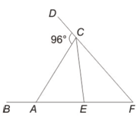(a) 58

(b) 64

(c) 72

(d) 32

8) If length of each side of a rhombus ABCD is 16 cm and ∠ABC = 120o, then what is the length (in cm) of BD?

(a) 24

(b) 12

(c) 16

(d) 14√3

9) In an isosceles triangle PQR, ∠P = 130o. If I is the in-centre of the triangle, then what is the value (in degrees) of ∠QIR?

(a) 130

(b) 120

(c) 155

(d) 165

10) In the given figure, O is the centre of a circle of radius 13 cm and AB is a chord perpendicular to OD. If CD = 8 cm, then what is the length (in cm) of AB?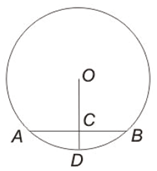(a) 6 cm

(b) 12 cm

(c) 24 cm

(d) 28 cm

x² – 7x + 1 = 0

x – 7 + 1/x = 0                     (Multiply the equation by 1/x  )

x + 1/x = 7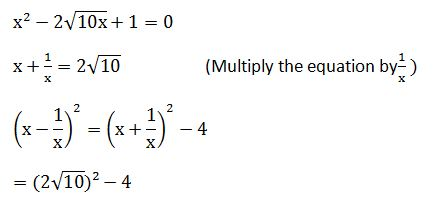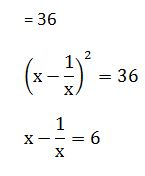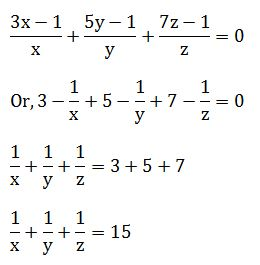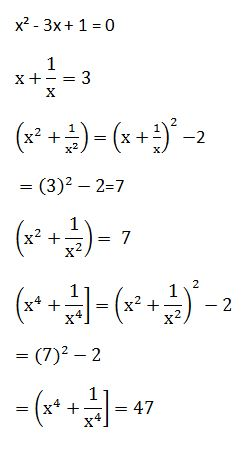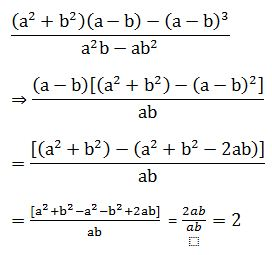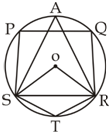∠SAR = 180° – ∠STR                      [Cyclic quadrilateral]

= 180° – 105°

= 75°

∠SOR = 2∠SAR

= 150°

Now,

∠OSR = ∠ORS = x [OS = OR = r(radius]

∠OSR =1/2  (180 – 150)

= 15°

∠PSR = 180 – ∠PQR (cyclic quadrilateral)

= 180° – 100°

= 80°

∠OSP = ∠PSR – ∠OSR

= 80° – 15°

= 65°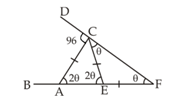Let ∠CFE= θ

∵∠ECF = ∠EFC (∵EC = EF)

∴∠FCE = θ

∠CEA = 2θ  (by exterior angle theorem]

∠CEA = ∠CAE = 2θ  (∵ AC = EC)

Now,

In ∆ACF

∠CAE + ∠CFA = ∠ACD                  (by exterior angle theorem)

2θ + θ = 96

3θ = 96

θ = 32

∠EAC = 2θ = 64°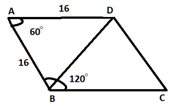= 60°

BD belongs to equilateral triangle ABD.

So, BD = 16 cm.